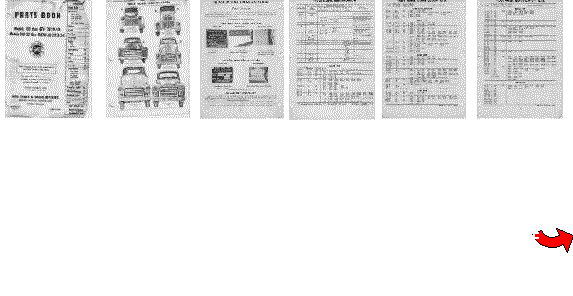First, get the chassis serial number off the ID Plate. GMC used a system to identify '39-'59 trucks by series, then wheelbase, then chassis number. It brakes down like this: From 1939 to 1950 they used letters, from '51-'53 numbers, then in ' 54 numbers followed by a letter The First letter is the "year bracket" ( A='39-'40, C='41-'46, E='46, F='47-'50 Z=54, Y='55 , X='56 , T=57, S='58/'59, N='60 ). The Second letter ('39-'50) is the cab type ( C = Conventional ) The Third and fourth digits are the series ( 10 = 1/2 ton-100 Series, 15 = 3/4 ton-150 Series, 25 = 1 ton-250 series, 28 =280, 30 = 300 and so on) The fifth digit (or third digit in '51-'53) is the series is the wheelbase (such as in 1/2 through 1 ton, 1=116", 2=125.25", and 3=137" in '47-'54) The sixth through twelfth digits are the regular production numbers which are simply a sequential number given to each truck as it came off the line. There are also muliple letter codes for larger trucks indicating a COE, tandem axle, or diesel engine. GMC didn't really look at truck "years" so much as at did model runs which in many cases ran for multiple years. The chassis serial number for each year are only found through multiple sources and there is no central registry of GMC serial numbers (also incorrectly called VINs) other than what I have put here as far as I know. The numbers, for the most part, started at 101 in '47 and went up from there for each individual series. So the first 1/2 ton off the line in '47 was FC101 001 and the first 3/4 ton was FC152 001. In the mid 50s, they started each year with 1001. If your serial is something like " 102 22 P 2537 " then your truck is: 10 =1/2 ton, 2 =longbed, 22 =228 engine (24=248 engine), P =Pontiac, Michigan assembly (C = Oakland, CA and S=St. Louis, MO), 2537 =regular production number which would by the charts below make this truck a 1951 longbed halfton (1001-28735 was production for '51 in 1/2 ton trucks for a total of 27734 trucks. #2537 would have been the 1536th 1/2 ton off of the Pontiac line in '51. Use the pages below and read them from left to right using the continue button on lower right corner of each page.